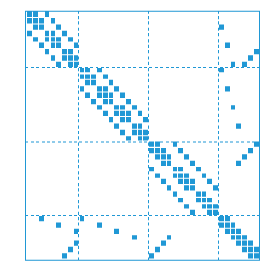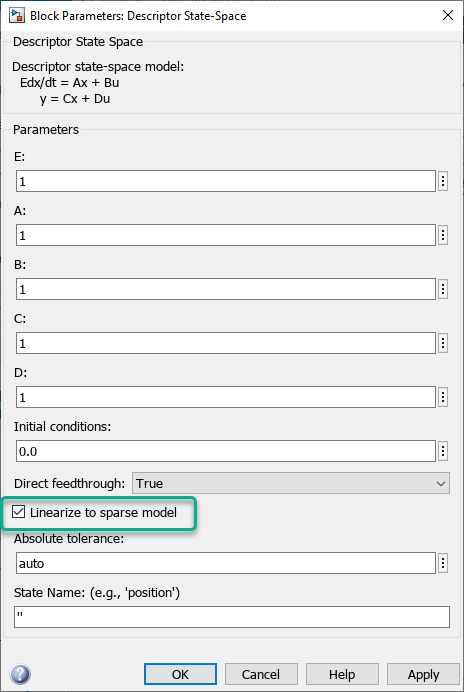## Sparse Model Basics

### Model Objects

Use the `sparss` and `mechss` objects to represent sparse first-order and second-order systems, respectively. Such sparse models arise from finite element analysis (FEA) and are useful in fields like structural analysis, fluid flow, heat transfer and electromagnetics. FEA involves analyzing a problem using finite element method (FEM) where a large system is subdivided into numerous, smaller components or finite elements (FE) which are then analyzed separately. These numerous components when combined together result in large sparse models which are computationally expensive and inefficient to be represented by traditional dense model objects like `ss`.

Sparse matrices provide efficient storage of double or logical data that has a large percentage of zeros. While full (or dense) matrices store every single element in memory regardless of value, sparse matrices store only the nonzero elements and their row indices. For this reason, using sparse matrices can significantly reduce the amount of memory required for data storage. For more information, see Computational Advantages of Sparse Matrices.

The following table illustrates the types of sparse models that can be represented:

Model TypeMathematical RepresentationModel Object
Continuous-time sparse first-order model

`sparss`
Discrete-time sparse first-order model

`sparss`
Continuous-time sparse second-order model

`mechss`
Discrete-time sparse second-order model

`mechss`

You can use `sparssdata` and `mechssdata` to access the model matrices in sparse form. You can also use the `spy` command to visualize the sparsity of both first-order and second-order model matrices.

You can also convert any non-FRD model into a `sparss` or a `mechss` object respectively. Conversely, you can use the `full` command to convert sparse models to dense storage `ss`. Converting to dense storage is not recommended for large sparse models as it result in high memory usage and poor performance.

### Combining Sparse Models

#### Signal-Based Connections

All standard signal-based connections listed under Model Interconnection are supported for sparse model objects. Interconnecting models using signals allows you to construct models for control systems. You can conceptualize your control system as a block diagram containing multiple interconnected components, such as a plant and a controller connected in a feedback configuration. Using model arithmetic or interconnection commands, you combine models of each of these components into a single model representing the entire block diagram.

In series and feedback connections of `sparss` models, eliminating the internal signals can lead to undesirable fill-in in the `A` matrix. To prevent this, the software eliminates only those signals that do not affect the sparsity of `A` and adds the remaining signals to the state vector. In general, this produces a differential algebraic equation (DAE) model of the interconnection where the `A` matrix has a block arrow structure as depicted in the following figure:Here, each diagonal block is a sub-component of the sparse model. The last row and column combines the `Interface` and `Signal` groups to capture all couplings and connections between components.

The same rules apply to second-order `mechss` model objects. You can use `showStateInfo` to print a summary of the state vector partition into components, interfaces, and signals

Use the `xsort` command to sort states based on state partition. In the sorted model, all components appear first, followed by the interfaces, and then followed by a single group of all internal signals.

For examples, see the `sparss` and `mechss` reference pages.

#### Physical Coupling

You can use the `interface` command to specify physical couplings between the components of a `mechss` model. `interface` uses dual assembly formulation where the global set of degrees of freedom (DoFs) is retained and an assembly is made by describing coupling constraints at the interface. For rigid couplings between DOFs N1 of substructure S1 and DOFs N2 of substructure S2, these include:

• Displacement matching: q(N1) = q(N2)

• Action/reaction principle: g(N1) + g(N2) = 0 where g(N1) are the forces exerted by S2 on S1, and g(N2) the forces exerted by S1 on S2.

These constraints can be summarized by the equations:

where g is the vector of the interface forces and H is a suitable DOF-selecting matrix.

You can also specify non-rigid couplings between the DOFs using the `interface` command.

For more information, see `interface` and Rigid Assembly of Model Components.

#### Combining Models of Different Types

The following precedence rules apply when combining models of different types:

For examples, see the `mechss` reference page.

### Time-Domain Analysis

All standard time-domain analysis functions listed under Time and Frequency Domain Analysis are supported for `sparss` and `mechss` model objects.

You must specify a final time or time vector when using time-domain response functions for sparse models. For example:

```tf = 10; step(sys,tf) t = 0:0.001:1; initial(sys,x0,t)```

The time response functions rely on custom fixed-step DAE solvers that have been developed especially for large-scale sparse models. You can configure the DAE solver type and enable parallel computing by using the `SolverOptions` property of the `sparss` and `mechss` model objects. Parallel computing can be used to accelerate `step` or `impulse` response simulation for multi-input models as the response for each input channel is simulated in parallel. Enabling parallel computing requires a Parallel Computing Toolbox™ license.

The available solver options are outlined in the table below:

DAE SolverDescriptionUsage
`'trbdf2'`Fixed step solver with accuracy of `o(h^2)`, where `h` is the step size. `'trbdf2'` is 50% more computationally expensive than `'hht'`. This is the default DAE solver option for both `sparss` and `mechss` models.Available for both `sparss` and `mechss` models.
`'trbdf3'`Fixed step solver with accuracy of `o(h^3)`. `'trbdf3'` is 50% more computationally expensive than `'trbdf2'`.Available for both `sparss` and `mechss` models.
`'hht'`Fixed step solver with accuracy of `o(h^2)`, where `h` is the step size. `'hht'` is the fastest but can run into difficulties with high initial acceleration like the impulse response of a system.Available for `mechss` models only.

To enable parallel computing and solver selection, use the following syntax:

```sys.SolverOptions.UseParallel = true; sys.SolverOptions.DAESolver = 'trbdf3';```

For an example, see Linear Analysis of Cantilever Beam.

### Frequency-Domain Analysis

All standard frequency-domain analysis functions listed under Time and Frequency Domain Analysis are supported for `sparss` and `mechss` model objects. For frequency response computations, enabling parallel computing speeds the response computation by distributing the set of frequencies across available workers. Enabling parallel computing requires a Parallel Computing Toolbox license.

You must specify a frequency vector when using frequency response functions for sparse models. For example:

```w = logspace(0,8,1000); bode(sys,w) sigma(sys,w)```

For an example, see Linear Analysis of Cantilever Beam.

### Continuous and Discrete Conversions

You can convert between continuous-time and discrete-time, and resample `sparss` models using `c2d`, `d2c` and `d2d`. The following table outlines the available methods for `sparss` models:

MethodDescriptionUsage
`'tustin'`Bilinear Tustin approximationAvailable with `c2d`, `d2c` and `d2d` functions.
`'damped'`Damped Tustin approximation based on TRBDF2 formula. This method provides damping at infinity in contrast to the `'tustin'` method, that is, the high frequency dynamics are filtered out and do not contribute to an accumulation of modes near `z = -1` in the discrete model (a source of numerical instability).Available with `c2d` only.

Note

Currently, `mechss` models are not supported for continuous and discrete conversions. Convert to `sparss` model form for discretization.

### Sparse Linearization

You can obtain a sparse linearized model from a Simulink® model when a Descriptor State-Space (Simulink) or Sparse Second Order block is present.

• The Sparse Second Order block is configured to always linearize to a `mechss` model. As a result, the overall linearized model is a second-order sparse model when this block is present.

• Check the Linearize to sparse model option in the Descriptor State-Space (Simulink) block to linearize to a `sparss` model. You can also achieve this by setting the `LinearizerToSparse` parameter to `'on'` in the Descriptor State-Space (Simulink). By default, the Linearize to sparse model box is unchecked and the `LinearizerToSparse` is `'off'`. The block-level `LinearizeToSparse` setting is ignored when you specify a replacement for the block, either with the `SCDBlockLinearizationSpecification` block parameter, or the `blocksub` input to `linearize` (Simulink Control Design).This linearization workflow requires Simulink Control Design™ software.

For an example, see Linearize Simulink Model to a Sparse Second-Order Model Object.

#### Linearize Structural or Thermal PDE Model

You can obtain a sparse linearized model from a structural or a thermal PDE model by using the `linearize` (Partial Differential Equation Toolbox) function.

• For a structural analysis model, `linearize` extracts a `mechss` model.

• For a thermal analysis model, `linearize` extracts a `sparss` model.

Use `linearizeInput` (Partial Differential Equation Toolbox) to specify the inputs of the linearized model in terms of boundary conditions, loads, or internal heat sources in the PDE model. Use `linearizeOutput` (Partial Differential Equation Toolbox) to specify the outputs of the linearized model in terms of regions of the geometry, such as cells (for 3-D geometries only), faces, edges, or vertices.

This linearization workflow requires Partial Differential Equation Toolbox™ software.

For examples, see Linear Analysis of Cantilever Beam and Linear Analysis of Tuning Fork.

### Other Supported Functionality

Additionally, the following functionality is currently supported for sparse models:

### Limitations

The following operations are currently not supported for sparse models:

• Pole/zero and stability margin computation

• Compensator design and tuning

• Model order reduction

You can use the `full` command to convert small sparse models to dense storage `ss` to perform the above operations. Converting to dense storage is not recommended for large sparse models as it may saturate available memory and cause severe performance degradation.

The following limitations exist for sparse linearization:

• When you set the `linearize` (Simulink Control Design) option `BlockReduction` to `'off'`, the model is always linearized to a dense `ss` model. This is because block substitution is disabled in this case.

• Sparse linearization is incompatible with block substitutions involving tunable or uncertain models. The Linearize to sparse model option should be unchecked when trying to linearize to `genss` or `uss` (Robust Control Toolbox) models.

• Snapshot linearization may not work when the Descriptor State-Space (Simulink) or Sparse Second Order block is present. Since snapshot linearization requires simulation, the simulation capabilities are currently limited for the Descriptor State-Space (Simulink) and Sparse Second Order blocks.

 H. Hilber, T. Hughes & R. Taylor. " Improved numerical dissipation for time integration algorithms in structural dynamics." Earthquake Engineering and Structural Dynamics, vol. 5, no. 3, pp. 283-292, 1977.

 M. Hosea and L. Shampine. "Analysis and implementation of TR-BDF2." Applied Numerical Mathematics, vol. 20, no. 1-2, pp. 21-37, 1996.